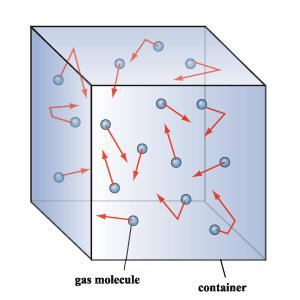# EXPLAINING BROWNIAN MOTION

 Molecular Kinetic Theory of Heat In this theory for describing phenomenon, gases are modeled AS IF they are composed of tiny particles. You should note that at NO time did the scientists working on this theory claim that matter was actually composed of atoms, they simply modeled gases as if they were. There are some basic assumptions that are made by this theory. They are: 1. A macroscopic volume contains a large number of these tiny particles. This means that for a volume that we would see every day, like the amount of water in a glass. there are on the order of a million billion billion of these tiny particles. The exact number is known as Avogadro's number and will be discussed in detail in following pages. 2. The seperation between the particles is large. You might say how can this be if there as an Avogadro's number of particles in a volume. When we refer to large in this assumption, we are comparing it to the size of the particles themselves. So the spacing between the particles is large when compared with the actual size of the particles. 3. There are no forces between the molecules expect when they collide. This basically means that the particles are not attracted to each other in any way that will effect their motion. The only time that they experience a force is when they are struck by other particles. 4. The collisions are elastic. This basically means that when any two particles collide both the momentum and the energy are conserved. For example, if both particles are moving at a speed of 20m/s and they collide head on, then they bounce backwards and return in the opposite direction moving at the same speed. 5. The directions of the particles velocities are random. This means that we are just as likely to find a specific particle moving in any given direction. There is NO preferred direction. The figure below gives an example of the type of model they were describing in this theory.The following site contains an excellent summary of all areas of thermodynamics. Please see the HYPER PHYSICS SITE.